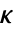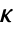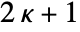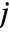AtomicDensityMatrix`
AtomicDensityMatrix`

# Rank

Rank[tens]

returns the tensor rankof irreducible spherical tensor tens.

Rank[op]

returns the angularmomentum quantum numbercorresponding to the dimension of operator op.

Rank[decomp]

returns the list of ranks of the tensors making up a sphericaltensor decomposition decomp.

Rank[mat]

returns a matrix of lists of ranks for the decompositions making up a tensordecomposition matrix mat.

# Details and Options

• A rank-spherical tensor hascomponents.
• Rank assumes that a supplied operator acts on a rank-angular-momentum space. A rank-operator is amatrix.
• Rank returns the rank of the top-level tensor for nested tensor structures.

# Examples

## Basic Examples(1)

Rank of a contravariant spherical tensor:

Of a covariant spherical-tensor operator:

Of an operator:

Of a contravariant spherical tensor decomposition:

Of a tensor decomposition matrix: